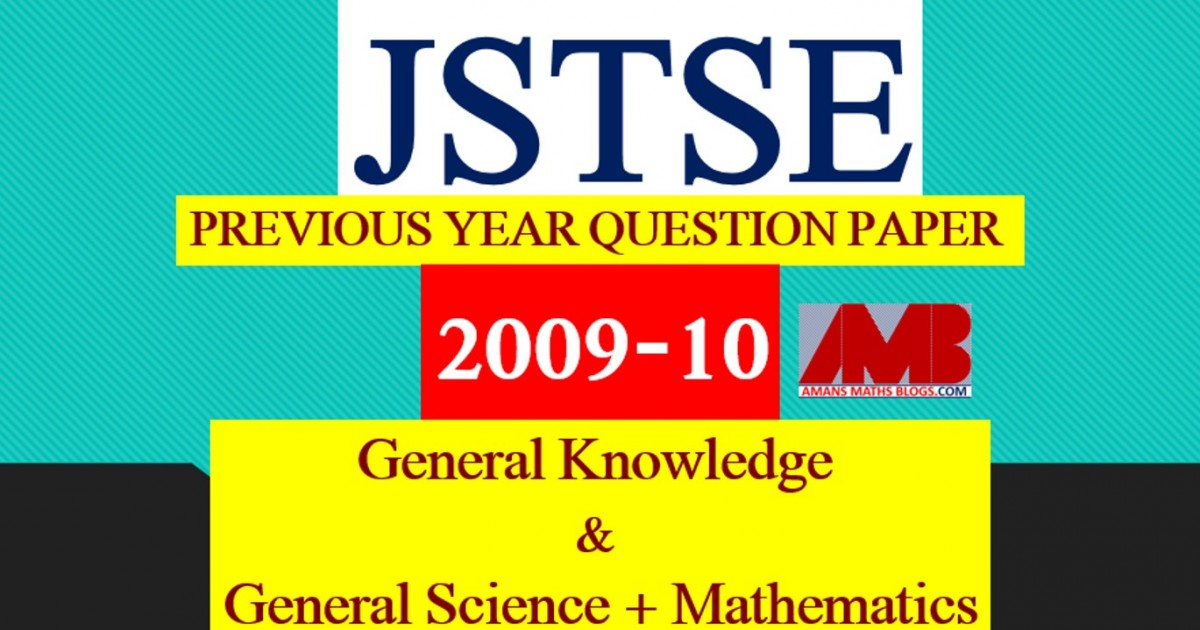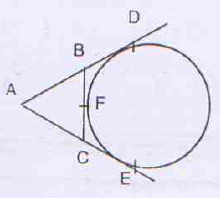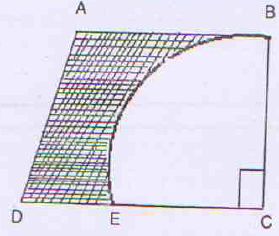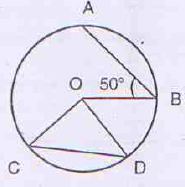Saturday, June 12, 2021
Home > download jstse papers > JSTSE PREVIOUS YEAR QUESTION PAPER 2009-10

# JSTSE PREVIOUS YEAR QUESTION PAPER 2009-10# JSTSE PREVIOUS YEAR QUESTION PAPER 2009-10

In this post, you will get JSTSE Previous Year Question Paper 2009-10.

Junior Science Talent Search Examination (JSTSE) is conducted by Science Branch of Dte. Of Education, Delhi every year usually in the month of January for the students of recognized schools of Delhi.
Eligibility: – Any student of recognised school studying in Class IX in Govt./Govt. Aided/Public/KV/Navodaya/NDMC and must have secured 65% marks in class VIII.

JSTSE
Previous Year Question Papers
2007-082008-092009-102010-112011-12
2012-132013-142014-152015-162016-17
2018-19PhysicsChemistryMathsBiology

Examination fee: – No examination fee is charged from students for appearing in this exam.
Type of Examination: – The examination consists of two papers.
I. General Knowledge
No. of Questions: 50       Marks : 50     Time Alloted: 50 min
50 50 50 min.
II . General Science and Mathematics
No. of Questions : 150      Marks : 150     Time Alloted: 150 min

No. of Scholarships:- There are 150 scholarships for JSTSE per year.

## General Science + Mathematics

Click Here To Download This File

JSTSE Previous Year Question Papers 2009-10 Ques No 1:

The decimal expansion of 17/3125 will be

Options:

A. Terminating

B. Non-Terminating

C. Non-Terminating Repeating

D. None of the Above

JSTSE Previous Year Question Papers 2009-10 Ques No 2:

If x + 1/x = 4, then the value of x3 + 1/x3 will be

Options:

A. 54

B. 52

C. 32

D. 12

JSTSE Previous Year Question Papers 2009-10 Ques No 3:

If x = 1/(2 – √3), then the value of (x2  – 4x – √3) will be

Options:

A. 1 – √3

B. -1 + √3

C. -1 – √3

D. 2 + √3

JSTSE Previous Year Question Papers 2009-10 Ques No 4:

Simplifying (2n + 2n-1) / (2n+1 – 2n) will be

Options:

A. 1/2

B. 3/2

C. (1/2)(2n – 1)

D. (3/2)(2n + 1)

JSTSE Previous Year Question Papers 2009-10 Ques No 5:

If both x – 2 and x – 1/2 are factors of px2 + 5x + r then

Options:

A. p = r

B. p > r

C. p < r

D. none of the above

JSTSE Previous Year Question Papers 2009-10 Ques No 6:

If f(x) = ax2 + bx + c has no real roots and a + b + c < 0, then

Options:

A. c = 0

B. c > 0

C. c < 0

D. none of the above

JSTSE Previous Year Question Papers 2009-10 Ques No 7:

In the following diagram, the graph of the polynomial f(x) = ax2 + bx + c is shown, thenOptions:

A. a < 0, b < 0 and c > 0

B. a < 0, b < 0 and c < 0

C. a < 0, b > 0 and c > 0

D. a < 0, b > 0 and c < 0

JSTSE Previous Year Question Papers 2009-10 Ques No 8:

If am ≠ bl, then the system of the equations: ax + by = c and lx + my = n

Options:

A. has infinitely many solutions

B. has no solutions

C. has a unique solution

D. may or may or not have a solution

JSTSE Previous Year Question Papers 2009-10 Ques No 9:

If a and b can take values 1, 2, 3, 4, then the number of equations of the form ax2 + bx + 1 = 0 having real roots

Options:

A. 12

B. 10

C. 7

D. 6

JSTSE Previous Year Question Papers 2009-10 Ques No 10:

The first and last term of an AP are a and l respectively. If S is the sum of all terms of AP and common difference is given by (l2 – a2)/[k – (l + a)], then the value of k will be

Options:

A. S

B. 2S

C. 3S

D. None of above

JSTSE Previous Year Question Papers 2009-10 Ques No 11:

The value of tan1°tan2°tan3°tan4°….tan89° is

Options:

A. 1

B. -1

C. 0

D. None of above

JSTSE Previous Year Question Papers 2009-10 Ques No 12:

The value of (1 + tan2θ)/(1 + cot2θ) is

Options:

A. sec2θ

B. -1

C. cot2θ

D. tan2θ

JSTSE Previous Year Question Papers 2009-10 Ques No 13:

The ratio of the length of the pole and its shadow is 1 : √3. The angle of elevation of the Sun is

Options:

A. 90°

B. 60°

C. 45°

D. 30°

JSTSE Previous Year Question Papers 2009-10 Ques No 14:

The distance between the points (cosθ, sinθ) and (sinθ, -cosθ) is:

Options:

A. √3

B. √2

C. 2

D. 1

JSTSE Previous Year Question Papers 2009-10 Ques No 15:

A triangular region is formed in the first quadrant by the line y = 2, x = 6 and y = x. The area of this triangular region is

Options:

A. 4 sq units

B. 6 sq units

C. 8 sq units

D. 18 sq units

JSTSE Previous Year Question Papers 2009-10 Ques No 16:

If D, E, F are mid points of sides BC, CA and AB respectively of ∆ABC, then the ratio of areas the triangle DEF and ABC will be

Options:

A. 1 : 4

B. 1 : 2

C. 2 : 3

D. 4 : 5

JSTSE Previous Year Question Papers 2009-10 Ques No 17:

In the figure, if AD, AE and BC are tangents to the circle at D, E and F respectively, thenOptions:

A. AD = AB + BC + CA

B. 2AD = AB + BC + CA

C. 3AD = AB + BC + CA

D. None of above

JSTSE Previous Year Question Papers 2009-10 Ques No 18:

A solid is hemispherical at bottom and conical above. If the surface area of two parts are equal, then the ratio of its radius and its slant height of its conical part is

Options:

A. 1 : 3

B. √3 : 1

C. 1 : √3

D. 1 : 1

JSTSE Previous Year Question Papers 2009-10 Ques No 19:

A number is selected from numbers 1 to 27. The probability that it is a prime is:

Options:

A. 5/6

B. 1/6

C. 2/3

D. 1/3

JSTSE Previous Year Question Papers 2009-10 Ques No 20:

In the given figure, AB = BC = 7 cm and DE = 2 cm, then the shaded area of the figure isOptions:

A. 22.5 cm2

B. 38.5 cm2

C. 17.5 cm2

D. None of above

JSTSE Previous Year Question Papers 2009-10 Ques No 21:

In the given figure, O is the centre of the circle, then the value of x isOptions:

A. 43°

B. 65°

C. 108°

D. 115°

JSTSE Previous Year Question Papers 2009-10 Ques No 22:

AD is the median of ∆ABC and DE is the median of ∆ABD. If area of ∆ABC is 80 cm2, then the area of ACDE is

Options:

A. 66.3 cm2

B. 60 cm2

C. 40 cm2

D. None of above

JSTSE Previous Year Question Papers 2009-10 Ques No 23:

If 29x = 1, then x will be

Options:

A. Non Negative Integer

B. 0

C. Not Defined

D. None of above

JSTSE Previous Year Question Papers 2009-10 Ques No 24:

In the figure, AB = CD and ∠ABO = 50 degree then ∠COD isOptions:

A. 50°

B. 60°

C. 75°

D. 80°

JSTSE Previous Year Question Papers 2009-10 Ques No 25:

In the figure, OM ⊥ AB, O is centre, then AB isOptions:

A. 5 cm

B. 6 cm

C. 9 cm

D. 10 cm

JSTSE
Previous Year Question Papers
2007-082008-092009-102010-112011-12
2012-132013-142014-152015-162016-17
2018-19PhysicsChemistryMathsBiology
error: Content is protected !!